Trusted answers to developer questions
Trusted Answers to Developer Questions

Related Tags

machine learning
classification
statistics

# What is the VC dimension?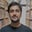Saqib Ali

Grokking Modern System Design Interview for Engineers & Managers

Ace your System Design Interview and take your career to the next level. Learn to handle the design of applications like Netflix, Quora, Facebook, Uber, and many more in a 45-min interview. Learn the RESHADED framework for architecting web-scale applications by determining requirements, constraints, and assumptions before diving into a step-by-step design process.

### Introduction

The Vapnik-Chervonenkis dimension, more commonly known as the VC dimension, is a model capacity measurement used in statistics and machine learning. It is termed informally as a measure of a model’s capacity. It is used frequently to guide the model selection process while developing machine learning applications. To understand the VC dimension, we must first understand shattering.

### Shattering

Shattering is the ability of a model to classify a set of points perfectly. More generally, the model can create a function that can divide the points into two distinct classes without overlapping. It is different from simple classification because it considers all possible combinations of labels upon those points. Later in the shot, we’ll see this concept in action while computing the VC dimension. In the context of shattering, we simply define the VC dimension of a model as the size of the largest set of points that that model can shatter.

### Find VC dimension

Let us consider a simple binary classification model, which states that for all points (a, b), such that a < x < b, label them as 1, otherwise, label them as 0.

$h(x)={1}$, if $a

$h(x)={0}$, otherwise

$(a, b) \in R^2$

We take two points, m and n. For these two points, there can be $2^2$ distinct labels in binary classification. We list these cases as follows:

$h(m)=0; h(n)=0$

$h(m)=0; h(n)=1$

$h(m)=1; h(n)=0$

$h(m)=1; h(n)=1$

We can observe that for all the possible labelling variations of mm and nn. The model can divide the points into two segments.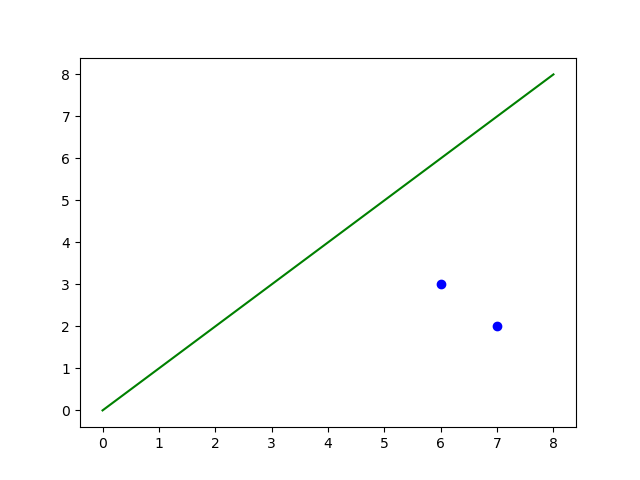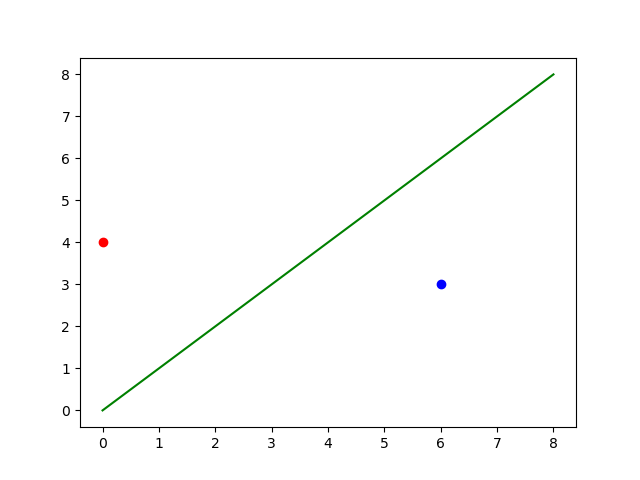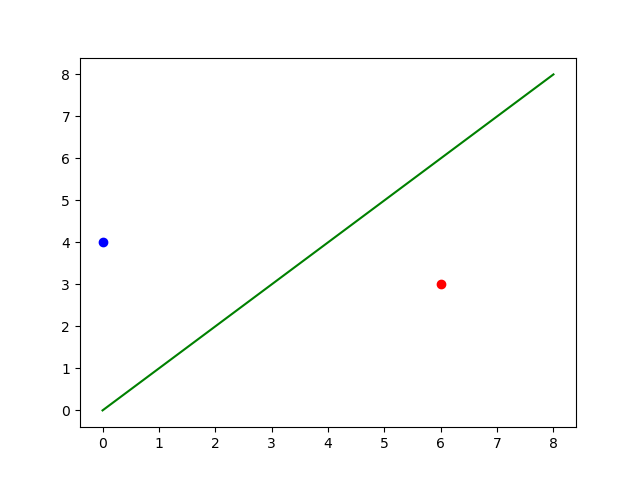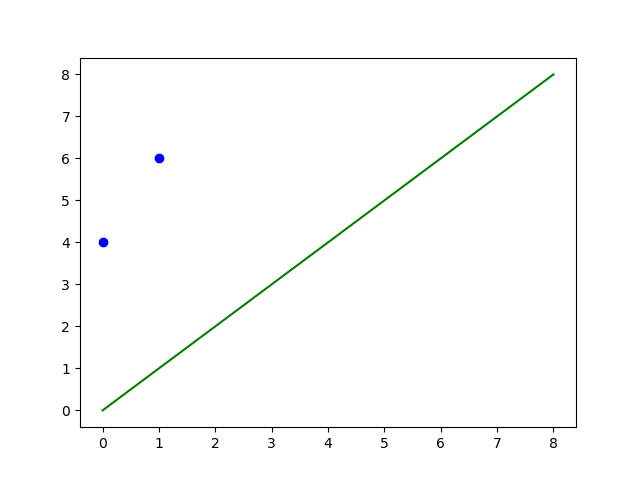This is where we can claim that our model successfully shattered two points in the dataset. Consequently, the VC dimension for this model is 2 (for now). Similar to the testing above, the modal also works on three points, which bumps our VC dimension to 3.

However, when we reach four points, we run into an issue. Specifically, in cases like these: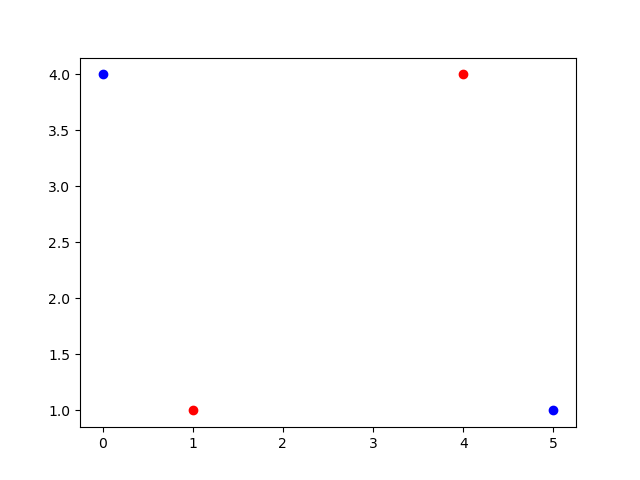There is no possible division through hyperplane in the plot above that can distinctly classify these points. Consequently, we can say that our shattering iteration failed, and our VC dimension is 3.

## Considerations & Keynotes

VC dimension is an essential metric in determining the capacity of a machine learning algorithm. It should be noted that the terms “capacity” and “accuracy” refer to two different things. The capacity of a model is defined as its ability to learn from a given dataset while accuracy is its ability to correctly identify labels for a given batch of data.

One model can have a high VC dimension but lower accuracy, and another model to have a low VC dimension but higher accuracy. It is also possible that a model with a high VC dimension is more likely to overfit the data, while a model with a low VC dimension is more likely to under fit the data.

Much like other metrics in machine learning, the VC dimension merely acts as a guiding light in model selection and shall be used with personal intuition.

RELATED TAGS

machine learning
classification
statistics

CONTRIBUTORSaqib Ali

Grokking Modern System Design Interview for Engineers & Managers

Ace your System Design Interview and take your career to the next level. Learn to handle the design of applications like Netflix, Quora, Facebook, Uber, and many more in a 45-min interview. Learn the RESHADED framework for architecting web-scale applications by determining requirements, constraints, and assumptions before diving into a step-by-step design process.

Keep Exploring

Learn in-demand tech skills in half the time